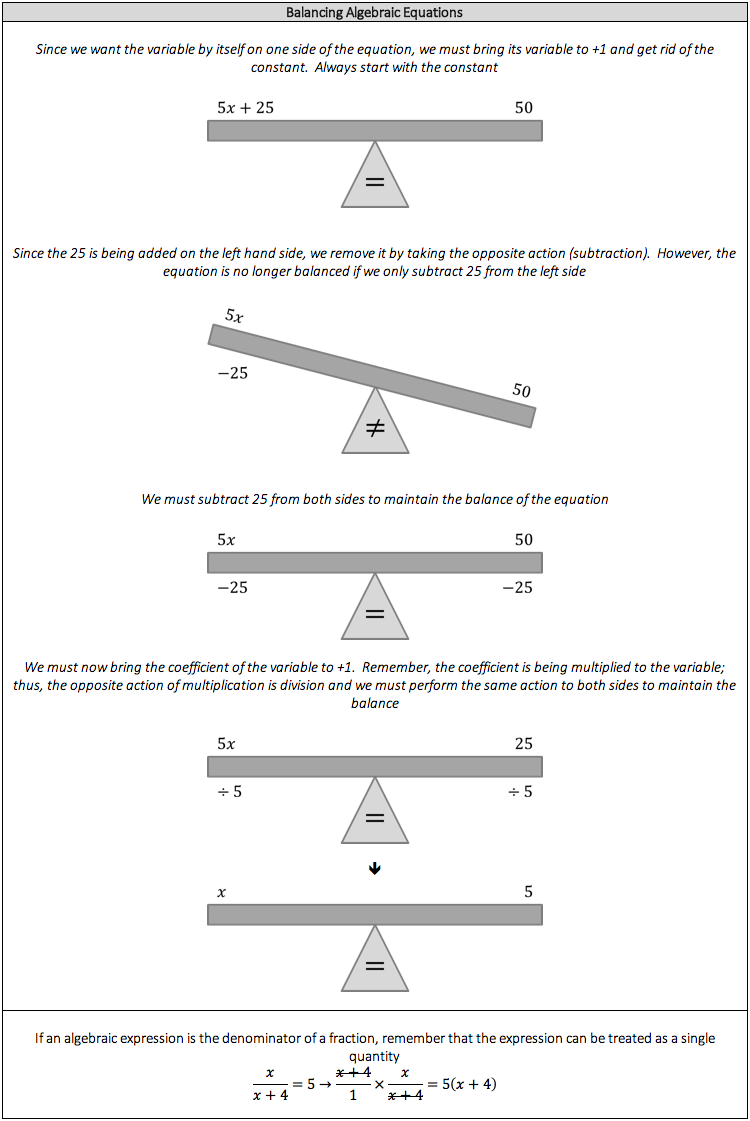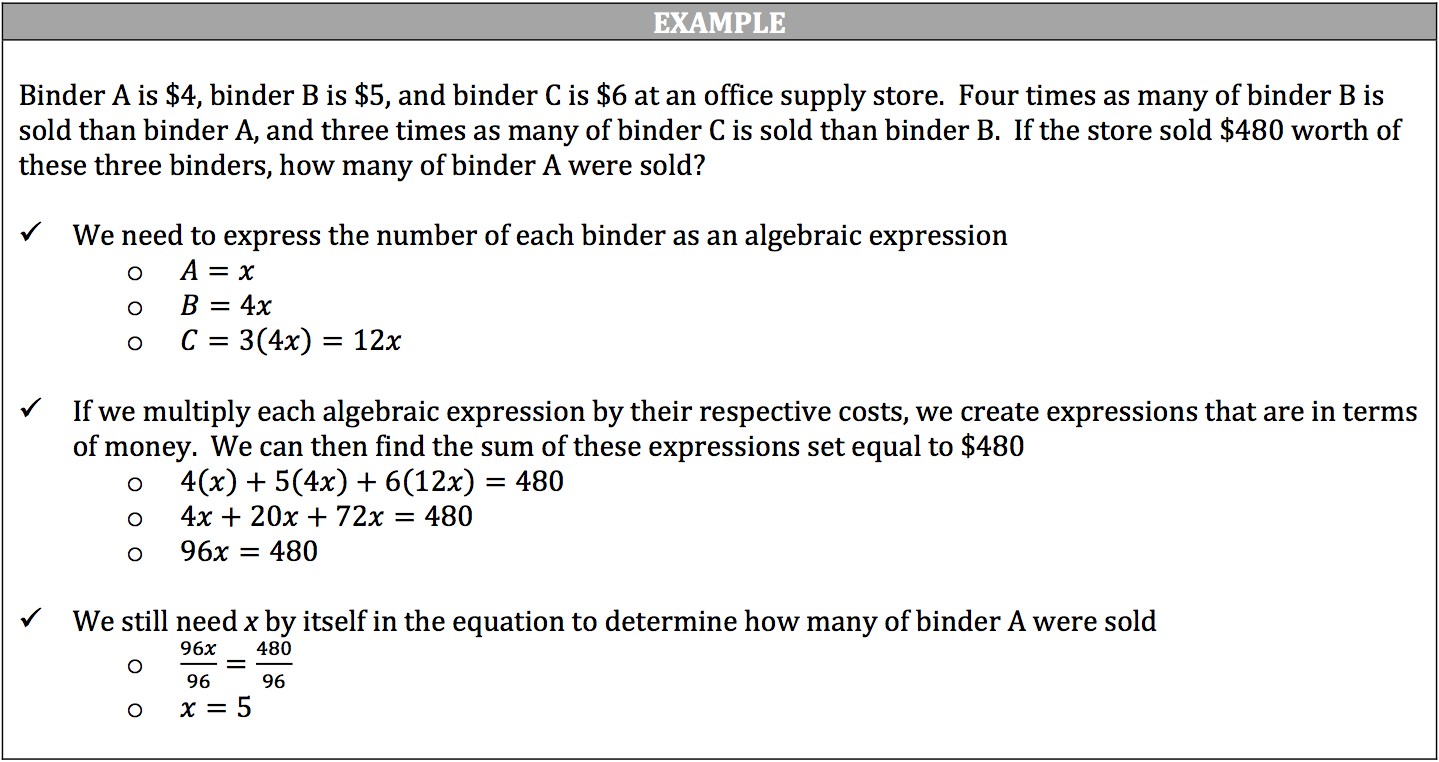The idea of the equation shouldn’t be new to you. We’ve already covered several concepts where an action results in a specific value. If you recall, there is always an equal sign between the action and the result, which is a statement that one expression is equal to the other. Since algebraic expressions can have multiple parts, we can manipulate an equation to make several mathematical statements.

Generally this means having a single variable on one side of the equation with everything else on the other side. Think of it in terms of a balanced seesaw where a person on one end of the seesaw weighs exactly the same as the person on the other end of the seesaw. If one person is handed a 10‐lb. weight, the balance is broken; however, the balance can be restored if the other person is handed a 10‐lb. weight. The same is true if we remove the 10‐lb. weight from one person and not the other. Long story short, whatever is done to one side of the equation must be done to the other side of the equation to maintain equivalence or balance.

### How to Balance Algebraic Equations